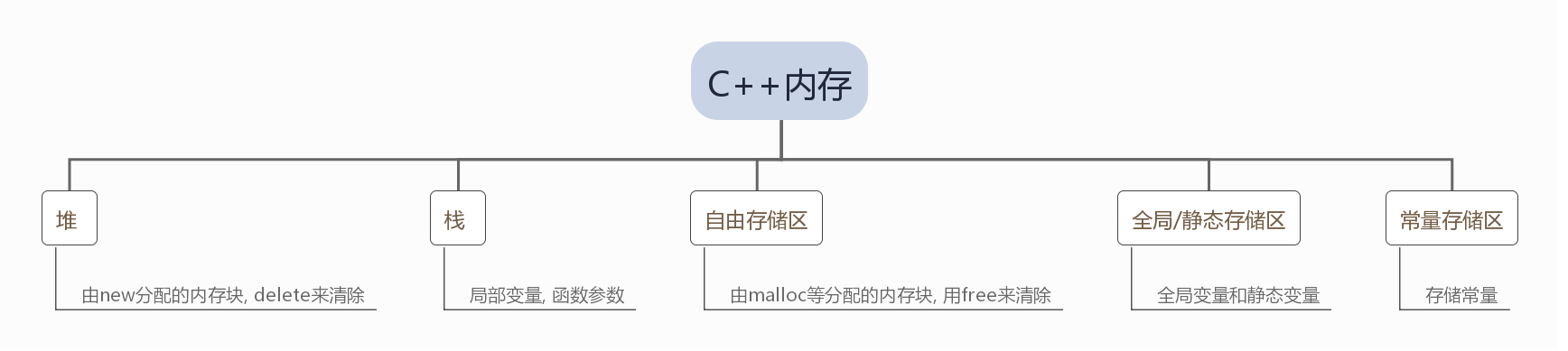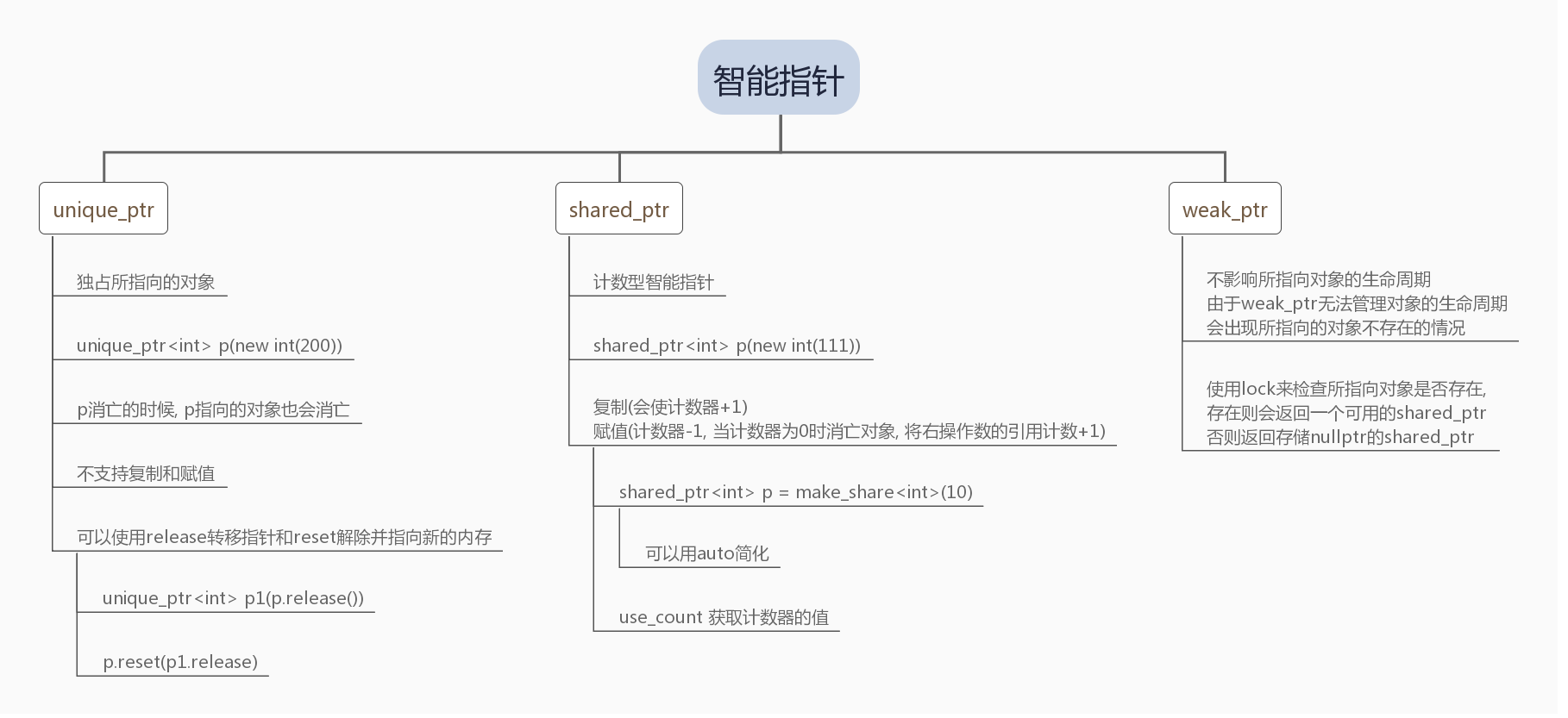# 定义新量

auto 自动根据初始值的类型进行自动类型推导.
decltype 根据表达式的类型定义对象

`std::move()`, 可以将一个

enum color {red, green, blue};

enum class color {red, green, blue};
`color a = color::red`

# 函数

• 直接传递数组长度
• 使用C风格字符串(默认结尾有标志)
• 使用C++11的新函数begin()和end()同时传递首尾地址

`bool (*pf)(int, int)` 可以指向
`bool max(int a,int b)`

Lambda
`[]() -> return type {statements}`

• 可以为空, 不会访问外围对象
• [=] 代表用值捕获的方式
• [&] 代表引用捕获

# 类

``````ostream &print(ostream &os)
{
os << 1 << endl;
return os;
}``````

C++ 中可以将该类函数声明为该类的友元.

C++11允许在显示定义构造函数的情况下使用默认构造函数

T(int a, int b):a_(a), b_(b){}

``````class A
{
int bar1_;
int bar2_;
A(int b1):A(b1, 2){}
A(int b1, int b2):bar1_(b1), bar2_(b2){}
}``````

T operator /(A, B);

``````// test.h
#include <cstdio>
class Test
{
public:
Test(int a, int b);
Test& operator+(const Test& right);
void PrintAB();
private:
int a_;
int b_;
};
// test.cpp
#include "test.h"
Test::Test(int a, int b)
{
a_ = a;
b_ = b;
}
Test& Test::operator+(const Test& right)
{
Test test(this->a_ + right.a_, this->b_ + right.b_);
return test;
}
void Test::PrintAB()
{
printf("%d %d\n", a_, b_);
}
// main.cpp
int main()
{
Test test1(1, 1);
Test test2(2, 2);
Test test = test1 + test2;
test.PrintAB();
return 0;
}

// ++ --
Test& operator++(); // 前置版本
Test operator++(int); // 后置版本``````

``````A T::*p1 = &T::x
int A::*p1 = &A::value
A a;
// 使用方式
a.*p = ......................;``````

p1指向T类中的 A类型的x数据成员
`A T::`可以用`auto`

``````int (A::*pf)();
pf = &A::GetValue;
// 当然可以使用auto 来简化
auto pf2 = &A::GetValue;
// 使用方式
(a.*pf)();
``````

`X x1 = {0} 和 X x2 {0}` 这两种是不同的实现!!, 大多数情况下这两个是相通的, 然而当
`explicit构造函数`存在的时候`前者的初始化是错误的`

`int x{0} int x= 0 int x(0)` 最后一个是错误的可能会和函数声明冲突

``````class A
{
typedef int x;
int z(x);
}``````

``````std::atomic<int> a1{0}; // OK
std::atomic<int> a2(0); // OK
std::atomic<int> a3 = 0; // Error``````

`std::atomic<int> a3 = 0``int类型`赋值到`可能是 cv-qualified``class type`
cv-qualified

``````// ***cv-qualified***
// non cv_qualified
int first;
char *second;

// cv-qualified
const int third;
volatile char * fourth; ``````

`std::atomic<int> a3 = 0` 满足了这个条件属于`copy-initialization`
copy-initialization

``````class A
{
public:
A () { } //直接初始化会调用这个构造函数
A (const A& a) { } //复制初始化会调用这个
}``````

`std::atomic<int> a3 {0}`这个会直接调用接收int的构造函数

`explicit`可以防止在调用某个成员函数的时候, 实参类型(通过构造函数之一)自动转换成相应参数,

@2019年10月26日21:25:58@算是第一次解决一个环环相扣的问题, 精力有点不足了
https://zhuanlan.zhihu.com/p/21102748 后续会继续进行理解

# 模板, 泛型 动态内存, 数据结构

``````template <typename T>
const T& GetMax(const T& a, const T& b)
{
return a > b ? a : b;
}
int main()
{
std::cout << GetMax(1, 2);
std::cout << GetMax<int>(1, 2); // 显示指定模板类型
return 0;
}``````C++堆的内存需要手动new和delete, 如果一个对象new出来没有在能被释放的时候释放就会造成–内存泄漏(需要及时delete不需要的对象)